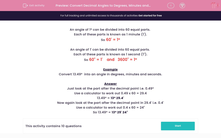# Convert Decimal Angles to Degrees, Minutes and Seconds

In this worksheet, students revise conversion of angles given in decimal format to angles in degrees, minutes and seconds.This content is premium and exclusive to EdPlace subscribers.Key stage:  KS 2

Curriculum topic:   Maths and Numerical Reasoning

Curriculum subtopic:   2D Shapes: Circles, Angles and Bearings

Difficulty level:#### Worksheet Overview

An angle of 1º can be divided into 60 equal parts.

Each of these parts is known as 1 minute (1').

So 60' = 1º

An angle of 1' can be divided into 60 equal parts.

Each of these parts is known as 1 second (1").

So 60" = 1'    and   3600" = 1º

Example

Convert 13.49º  into an angle in degrees, minutes and seconds.

Just look at the part after the decimal point i.e. 0.49º

Use a calculator to work out 0.49 x 60 = 29.4

13.49º = 13º 29.4'

Now again look at the part after the decimal point in 29.4' i.e. 0.4'

Use a calculator to work out 0.4 x 60 = 24"

So 13.49º = 13º 29' 24"

### What is EdPlace?

We're your National Curriculum aligned online education content provider helping each child succeed in English, maths and science from year 1 to GCSE. With an EdPlace account you’ll be able to track and measure progress, helping each child achieve their best. We build confidence and attainment by personalising each child’s learning at a level that suits them.

Get started